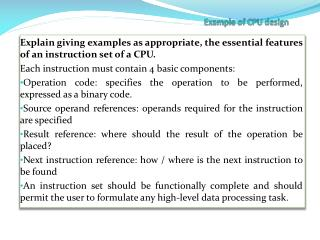DownloadDownload PresentationExample of CPU design

# Example of CPU design

Download Presentation## Example of CPU design

- - - - - - - - - - - - - - - - - - - - - - - - - - - E N D - - - - - - - - - - - - - - - - - - - - - - - - - - -
##### Presentation Transcript

1. Example of CPU design Explain giving examples as appropriate, the essential features of an instruction set of a CPU. Each instruction must contain 4 basic components: Operation code: specifies the operation to be performed, expressed as a binary code. Source operand references: operands required for the instruction are specified Result reference: where should the result of the operation be placed? Next instruction reference: how / where is the next instruction to be found An instruction set should be functionally complete and should permit the user to formulate any high-level data processing task.

3. Explain briefly the steps that you would take to design an instruction set for a 16-bit CPU. • 1. Decide on the instructions that you want to support as given above. Describe these in RTL notation for reference. • 2. Allocate the number of bits that you need to accommodate all the instructions you identified in 1 above. i.e. for 16 instructions use 4 bits. • 3. Decide the addressing mode for the operand fetch and allocate the bits for this (i.e. direct/indirect use 1 bit. This stage could be incorporated in 2 above to satisfy the number of instructions supported. Technically this now gives 32 possible instructions and that may be too many. • 4. Allocate the rest of bit to the operand value

4. Decode the following code using the instruction set information provided below • 0F FF 60 05 23 33 71 02

5. Convert hexadecimal to binary0F = FF = 60 = 05 = 23 = 33 = 71 = 02 =

6. Convert hexadecimal to binary0F = 0000 1111FF = 1111 111160 = 0110 000005 = 0000 010123 = 0010 001133 = 0011 001171 = 0111 000102 = 0000 0010

7. Map 16 bits onto architecture diagram 0F = 0000 1111 FF = 1111 1111 => 60 = 0110 0000 05 = 0000 0101 => 23 = 0010 0011 33 = 0011 0011 => 71 = 0111 0001 02 = 0000 0010 =>

8. Map 16 bits onto architecture diagram 0F = 0000 1111 FF = 1111 1111 => 60 = 0110 0000 05 = 0000 0101 => 23 = 0010 0011 33 = 0011 0011 => 71 = 0111 0001 02 = 0000 0010 => 0000 1111 1111 1111 0110 0000 0000 0101 0010 0011 0011 0011 0111 0001 0000 0110

9. Convert opcode to instruction mnemonics 0000 1111 1111 1111 0110 0000 0000 0101 0010 0011 0011 0011 0111 0001 0000 0110

10. Convert opcode to instruction mnemonics and xplain what registers and memory are affected at each step 0000 1111 1111 1111 0110 0000 0000 0101 0010 0011 0011 0011 0111 0001 0000 0110 LDA 0FF AC<-0FF SUB 5 AC AC<AC-5 STA  M <-AC ;MEMORY LOCATION 333 STORES ACCUMULATOR VALUE WHICH IS CHANGING ACCORDING TO THE VALUE AC-5. JNZ  JUMP IF NOT ZERO to 102 i.e. loop while not zero